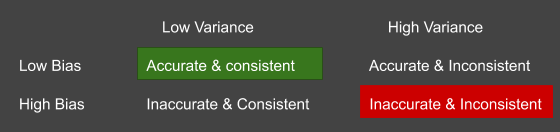• ## Scientists Using AI To Recreate Smells From 500 to 100 Years Ago

Introduction to bias, variance, bias-variance trade-off and its impact on the model.

As an absolute beginner in Machine Learning, some of the concepts might seem overwhelming. Bias and variance are among such concepts that often create confusion. It’s very important to understand such basic yet important concepts.

## Bias error

The term “Bias” means a preconceived opinion or strong inclination towards something. Same way, bias error is the error that results from assumptions (majorly, inaccurate) about the target function in a model. It occurs when assumptions about the mapping between input and output data are made, due to which algorithms have less flexibility to learn from the training set. Bias results in overlooking of features in data set hence not allowing the model to adapt to the training set fully.

Low Bias : Fewer assumptions about target function are made. KNN and decision tree can be considered as machine learning algorithms with low bias.

High Bias : More assumptions about target functions are made. Multiple Linear Regression and Logistic Regression can be considered as machine learning algorithms with high bias.

## Variance error

From the machine learning perspective, variance signifies the difference in fits between datasets. Variance error is the error that occurs when the model is very sensitive to the training data i.e. the model is strongly influenced by the specifics of the training data. This error occurs when numerous parameters of the target function depend strongly on the training data set and this further results in different estimates when the model is given new training data.

Low Variance : When changes in data-set result in small changes of the function estimate i.e. the fits of different data-sets do not vary much. Linear Regression can be considered as a machine learning algorithm with low variance.

High Variance : When changes in data-set result in large changes of the function estimates i.e. the difference between fits of data-sets is significant. Usually, algorithms with high complexity have high variance as such algorithms are free to learn any functional form from training data set.SVM and Decision tree can be considered as machine learning algorithms with high variance.

## Impact of variance and bias on model

Basically, bias is how far off predictions are from accuracy and variance is the degree to which the predictions vary between different realizations of the model.

Low variance algorithms tend to be consistent and simple structured with limited complexity. Such algorithms are faster to train.
Low bias algorithms tend to be accurate and flexible in structures with high complexity. Low bias algorithms are slower to train.
High variance algorithms learn random noise along with the underlying pattern from the training set which introduces inconsistency in the model. This often leads to overfitting.
High bias algorithms miss out important relations between features and output hence leading to underfitting. Predictions, in this case, are far off from correctness.Four different cases based on the level of variance and bias

For a good model, the total prediction error needs to be minimised.
Total Prediction error= Bias² + Variance + Irreducible error
Only model errors can be reduced hence bias error and variance error needs to be minimized.

Why is there a trade-off? Why can’t we have the best of both worlds?
Well, there is no way to avoid the relationship between bias and variance. It is quite challenging to minimize both the errors at the same time. Decreasing the bias will increase the variance and decreasing the variance will increase the bias. In simple terms, an increase in accuracy will lead to a decrease in consistency and vice versa.

The model needs to battle its way to find a balance between bias and variance. The model needs to settle somewhere in the middle of the complexity (highlighted by the dotted line in the below image) as a model cannot have high complexity(in case of low bias) and limited complexity (in case of low variance) at the same time.

1.adreamoftrains webhosting says: